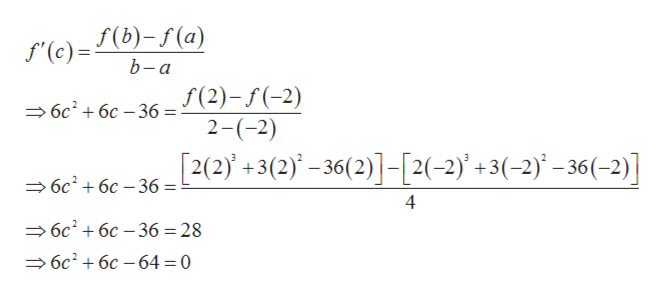# f(x)= 2x^3+3x^2-36xDetermine whether the Mean Value Theorem can be applied to f on [-2,2]. If the mean value theorem can be applied, find all value(s) of c in the open interval (-2,2) that satisfies the theorem.

Question
38 views

f(x)= 2x^3+3x^2-36x

Determine whether the Mean Value Theorem can be applied to f on [-2,2]. If the mean value theorem can be applied, find all value(s) of c in the open interval (-2,2) that satisfies the theorem.

check_circle

Step 1

Given,

Step 2

Since f is a polynomial in x, so it is continuous in the interval [-2, 2] and differentiable in the interval (-2, 2).

Now

Step 3

Now by mean value theorem there ex...help_outlineImage Transcriptionclosef(b)-f(a) f'(c)= b-а f(2)-(2) 2-(-2) — бс + бс —36 %— [2(2+3(2)-36(2)]-[2(-2)+3(-2) -36(-2)] 6c26c 36 = 4 6c26c 36 = 28 6c26c 64 0 fullscreen

### Want to see the full answer?

See Solution

#### Want to see this answer and more?

Solutions are written by subject experts who are available 24/7. Questions are typically answered within 1 hour.*

See Solution
*Response times may vary by subject and question.
Tagged in

### Calculus